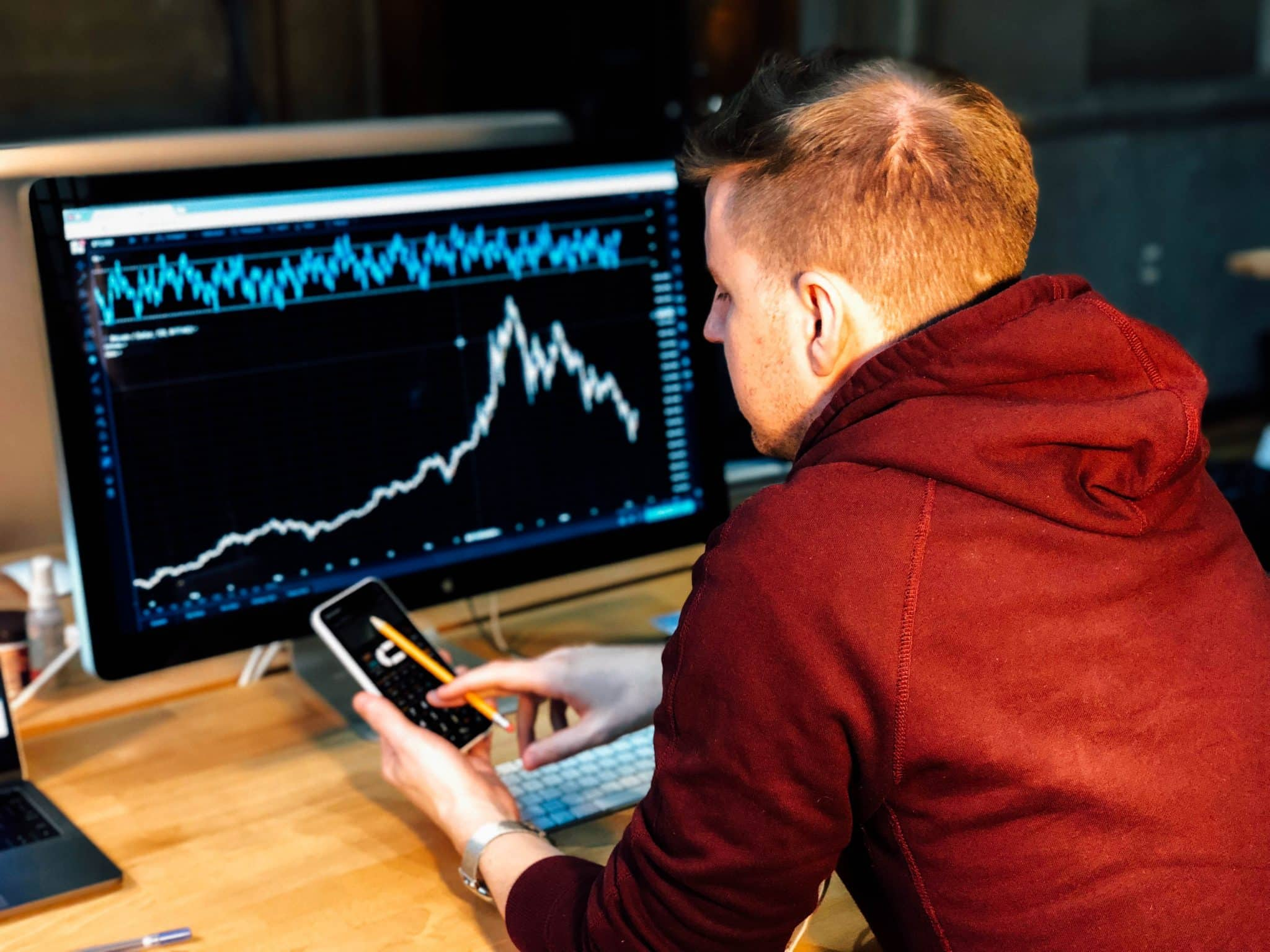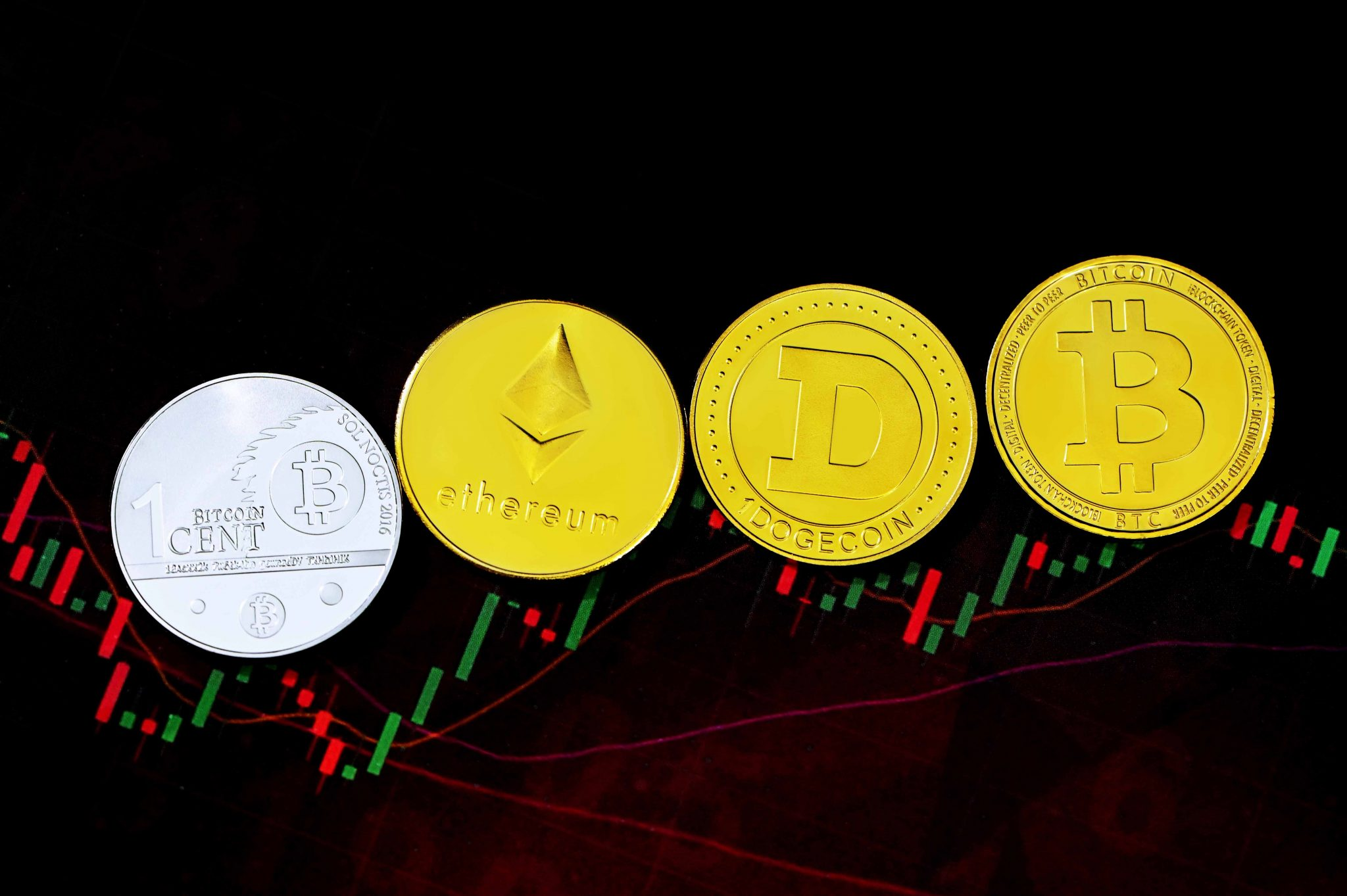Fibonacci is quite an exciting concept for traders. And the idea that every action has some reaction is entirely applicable here. To understand the concept of Fibonacci, you need to understand the concept in series. The concept of Fibonacci arose back in ancient times in Indian mathematics script in 200 BC.  The Fibonacci series follows 0,1,1,2,3,5,8,13,21,34…

Every number in the series is a sum of two preceding numbers, and the sequence goes on infinitely. The most remarkable thing about the Fibonacci series is that each number is 1.618 times greater than the other. The most common relationship between every number of the sequence is the psychology of forex trading and the base ratio used by technical traders to identify the retracement levels. The percentage of 61.8% determines the Fibonacci key, so found by dividing one number in the series by the following number. The 38.2% ratio is determined by dividing the number located two steps to the right in the series by the number followed.

The number three steps determine the 26.8% ratio to the right by the number that followed. 50% of Fibonacci retracements are generally included in the grid of charts shown in charting software. These levels are widely viewed with a strong potential in reversal levels. The concept of Fibonacci retracements is entirely subjective. Traders can use the technique in different ways. The profit-making from Fibonacci is very effective for the traders. The traders who suffer loss consider it unreliable. The technical analysis is self-fulfilling in Fibonacci retracements.

If the trader has a keen observation of the ratios, it will reflect in the price action in the technical indicator. The underlying principle of the Fibonacci tool does not have any grounded, logical proof. The formulas, integers, sequence, and everything on Fibonacci retracement depend upon the arrangement of the process.  The concept of Fibonacci is entirely unreliable. It is uncomfortable for traders who want to understand the reasoning behind the strategy.  The strategy of Fibonacci retracement can possibly meet the corrections and reversal countertrends.

Here the system struggles to determine the correctness of the information which is not easily identifiable.

## Is Fibonacci Retracement A Good Strategy?

Fibonacci strategies are the most trending strategy in the current times. The retracements in the charts go in the opposite direction of the trend. The trader here observes a low level of risk in the direction of movement. In order to use the Fibonacci trend, it is essential for you to understand and determine the Fibonacci moves ultimately. The actions can be in a 100% upward or downward trend.  To understand the trend moves, to mark 100, you are supposed to pick the highest peak through the chart. You need to get identified with the tools for connecting them. These tools are available in most of the technical identification tools in the packages.For instance, in EUR/USD, consider a few price points in different months of the year. Consider 4 points in April, May, June, July. Consider the downturn that began in April( spot1), and then the price again bottomed in may(spot2) and retraced at 38.2%.

Further, the Fibonacci levels go down and move towards spot 3. Here the 38.2% is a great spot to enter the position in order to capitalize on the continuation of the downtrend.  There are traders who are looking at 50% and 61.8%retracement levels in the market. Resuming the downtrend is majorly slow in fair price movements.  The reversals in the points here increase the convergence of technical indications when the price reaches a Fibonacci level. Other technical indicators include candlestick patterns, volume, momentum oscillators, moving averages. A more significant number of conforming indicators indicates more reversal signals.

These are used on a variety of instruments comprising stock, commodity, and currency exchange. These technical analyses are used on several time frames that predict the value in proportion to the used time. These timeframes are used to get greater weight in a longer time frame.

## What Are The Levels Of Fibonacci Retracement?

Fibonacci retracement levels are the sequence of numbers in the sum previous of two. These technical analyses predict future movements that identify the areas that bring balance to the assets. These charts are used to indicate the value of assets traded in a specific timeframe. These timeframes are recorded every minute, hours. Traders use these frameworks in different combinations for purposes.  The retracement levels in your chart are aligned with highs and lows in the markets. The high probability areas are the ideal times to enter and exit the market.

They are proven to have reverse price action after a new trend. Understanding different levels of Fibonacci retracements improves knowledge and market sentiments in vitality and trade sentiments.  Fibonacci retracement levels support resistance levels based on Fibonacci numbers. These numbers are 23.6%, 38.2%, 61.8% and 78.6%.

While drawing the Fibonacci levels, your software will include a 50% level of your nonofficial retracement levels. These levels are self-fulfilling proficiency. This allows the traders to have a keen observation of different groups that can be used during trading. These are the best trading support and resistance levels.  These lines never move straight, hence providing prominent price movement retractions. They are the most common type of technical indicator used by traders.

## What Is The Golden Ratio In Fibonacci?

There is always a unique ratio used to describe the nature of every small block in Fibonacci. The golden ratio refers to the predictable patterns of price in the retractable charts. These ratios are derived from the Fibonacci series. They are influenced by nature to maintain the balance in the financial market. The Fibonacci sequence is applied to the finances using several arcs, fans, time zones, and retracements.

Scientists have developed their golden ratios over the centuries. Italian scientist Leonardo Fibonacci derived it in the 1175 century A.D.   The sequence of every number in the Fibonacci is the sum of two preceding numbers. Here the series is not essential. The chain of quotients from the adjoining numbers here has a potent proportion. This is the inverse of 0.618 or a multiple of 1.618. Every number here holds a dimensional property in the ratio of 1.618 for the fundamental functioning of nature.  They are also called divine proportions. The higher numbers are close in relation to 1.168. They are represented by the greek letter phi Φ.

## How Do You Use The Fibonacci Indicator?

These indicators are also called retracements. Fibonacci retracements use different Fibonacci levels that indicate the prospective reversals in price in a solid upward trend. This means a period where the price moves above the trend. These movements last for two spots.  These indicators use numbers of spots pointed out by Leonardo Pisano. These indicators show the different percentages of retracements that show the Fibonacci series of numbers.   Every number here is a sum of two previous numbers in the series. For example, 0, 1, 1, 2, 3,5,8,13, 21 and so on.

You can plot different levels in the charts that represent the retracements from previous points. There are different levels of retracements in terms of depth. 38.2-50% of retracement levels are considered as average depth. 61.8% of retracement levels have higher depth and are considered as golden retracements levels.

### The Final ThoughtThe Fibonacci series is brought from Fibonacci retracements. These tools undergo the same problems as other universal strategies. Many traders use Fibonacci ratios to get success. The retracement in these trends uses long-term price trends. The formula for Fibonacci retracement might seem easy while analyzing. But they have a significant impact on the microanalysis of data. We hope we have given you a brief about Fibonacci retracement. Keep learning.

Also, Gather Interesting Information About Everything You Need To Know About Candlestick Patterns.

0 comment